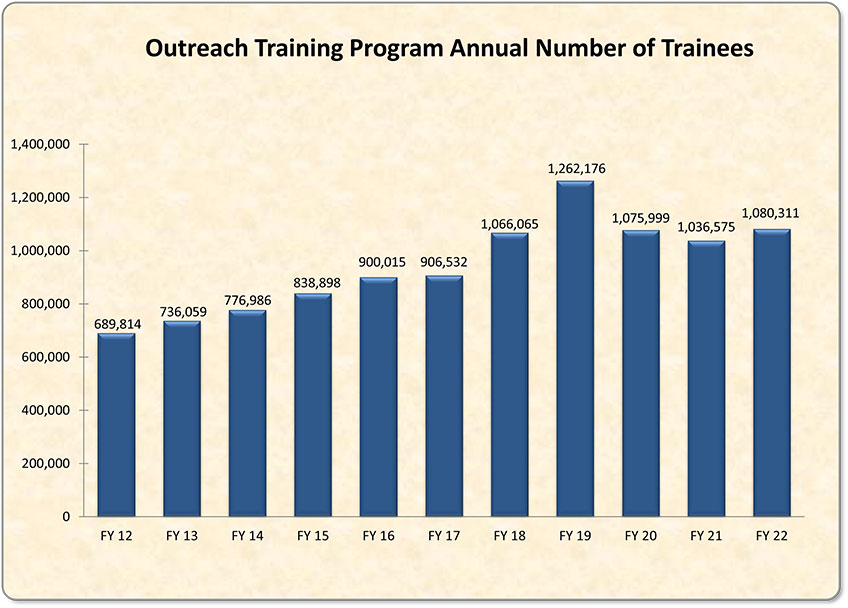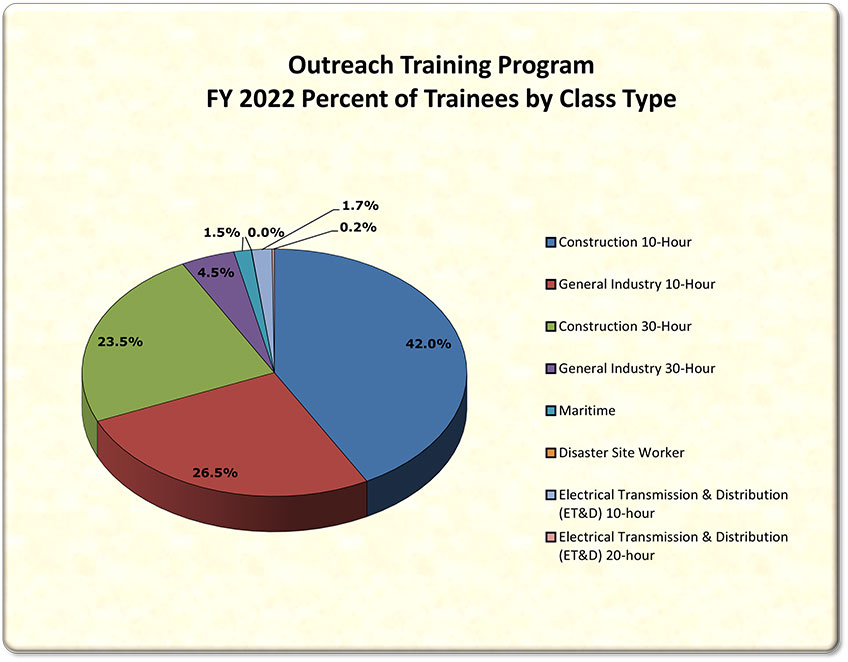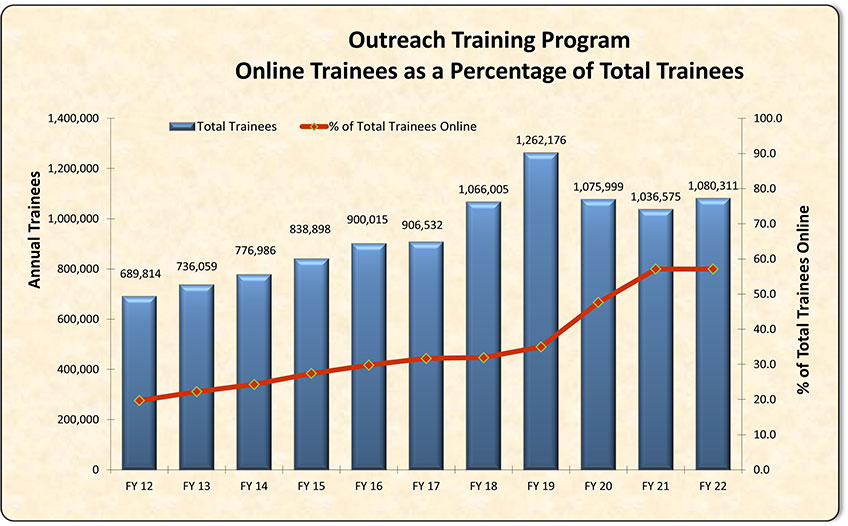### Outreach Training Program Growth#### Number of trainees in the last 5 years = 5.52 million

Text version of chart:
Title: Outreach Training Program Annual Number of Trainees
Chart Type: Vertical Bar Graph
Graph Elements: 11 - one bar for each fiscal year between to 2012 and 2022 showing the annual number of trainees.

Values:

• FY 2022 = 1,080,311
• FY 2021 = 1,036,575
• FY 2020 = 1,075,999
• FY 2019 = 1,262,176
• FY 2018 = 1,066,065
• FY 2017 = 906,532
• FY 2016 = 900,015
• FY 2015 = 838,898
• FY 2014 = 776,986
• FY 2013 = 736,059
• FY 2012 = 689,814

#### Number of Trainees by Class TypeText version of chart:
Title: FY 2022 Number of Trainees by Class Type
Chart Type: Pie Chart
Graph Elements: 8 segments by class type.

Values:

• Construction 10-Hour = 42.0%
• General Industry 10-Hour = 26.5%
• Construction 30-Hour = 23.5%
• General Industry 30-Hour = 4.5%
• Maritime = 1.5%
• Disaster Site = 0.0%
• Electrical Transmission & Distribution (ET&D) 10-Hour = 1.7%
• Electrical Transmission & Distribution (ET&D) 20-Hour = 0.2%

#### Outreach Training Program Online Trainees#### Number of trainees in the last 5 years = 2.48 million

Text version of chart:
Title: Outreach Training Program Online Trainees as a Percentage of Total Trainees
Chart Type: Vertical Bar Graph and Line Graph
Graph Elements: 22 - two bars for each fiscal year between 2012 and 2022 showing the number of online trainees and what percentage of the total number of trainees were trained online.

Values:

• FY 2022
• Online Trainees = 589,509
• % of Total Trainees = 54.6
• FY 2021
• Online Trainees = 592,239
• % of Total Trainees = 57.1
• FY 2020
• Online Trainees = 512,080
• % of Total Trainees = 47.6
• FY 2019
• Online Trainees = 441,978
• % of Total Trainees = 35.0
• FY 2018
• Online Trainees = 340,022
• % of Total Trainees = 31.9
• FY 2017
• Online Trainees = 287,789
• % of Total Trainees = 31.7
• FY 2016
• Online Trainees = 268,034
• % of Total Trainees = 29.8
• FY 2015
• Online Trainees = 230,001
• % of Total Trainees = 27.4
• FY 2014
• Online Trainees = 188,648
• % of Total Trainees = 24.3
• FY 2013
• Online Trainees = 163,106
• % of Total Trainees = 22.2
• FY 2012
• Online Trainees = 135,801
• % of Total Trainees = 19.7